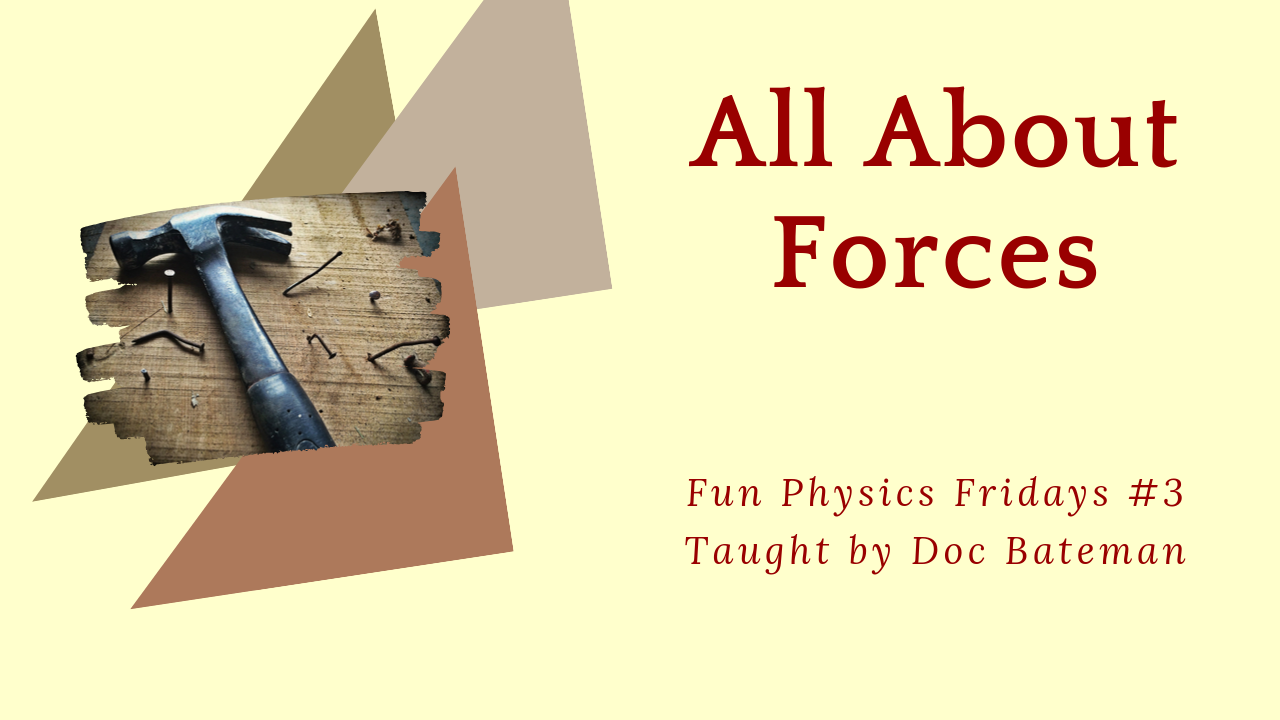## Forces: Fun Physics Friday #3

All about forces and Newton’s Second Law. Something that pulls or pushes on an object is exerting a force.

## Transcript

Hey there, Doc Bateman here. Today’s short video is about force. What do you know about force? It’s the subject of Newton’s Second Law and that’s what we’re going to talk about today. How can we use force to tell us other things about a system? Be sure to hit like and subscribe and don’t forget to get your free worksheet from my website, linked below. Let’s get started.

What is a force? Pushing or pulling on anything is called exerting a force. We’ll talk about several different ways to do this. Newton’s Second Law says that the sum of all forces on an object is equal to its mass times its acceleration (F = ma). If there is an unbalanced force in a particular direction, then the object accelerates in that direction.

If we heat a sealed container, then the heat causes the gas inside to expand, which increases the force on the inner walls of the container. Any object that has mass experiences a force due to gravity. As Newton said, force equals mass times acceleration. The force due to gravity is referred to as mg, or the mass times the acceleration of gravity, which we call g. The force of gravity is commonly called weight.

Forces have a magnitude and a direction; this is also called a vector, (a magnitude with a direction) and they can be applied in any direction. So when we add all the forces up, some forces can cancel out other forces. For example, if I push on the left side of a block by a certain amount, and then I push on the right side of the block by the same amount, then the sum of the forces is zero, and the block does not move. If our block is sitting on the floor, then gravity pulls down at a force equal to mg, and the floor pushes up on the block by the same amount. The block does not move up or down.

Forces can be applied continuously as pushing forces or instantaneously as a striking force. Think of hitting something with a hammer. Again according to Newton’s law, forces cause acceleration, so a pushing force is a constant acceleration a striking force would be an instantaneous acceleration. In the metric system, force is measured in newtons; in the imperial system, force is measured in pounds.

We can also apply a force to an object through an electric or magnetic field. To apply a magnetic force to an object, it has to be made of certain materials, like iron. To apply an electric force, one object has to have an electric charge.

Here’s my question: What do you think is happening here? Leave your answer for me in the comments below.

And now you should know all about forces. Leave an answer to my question in the comments below. Don’t forget to get you free worksheet over at my website. Thanks for watching.